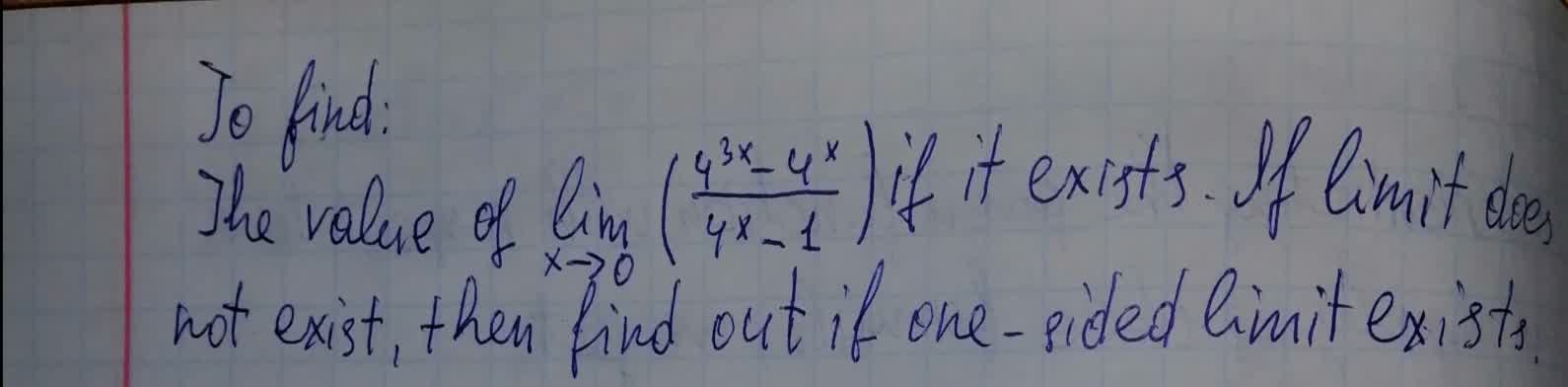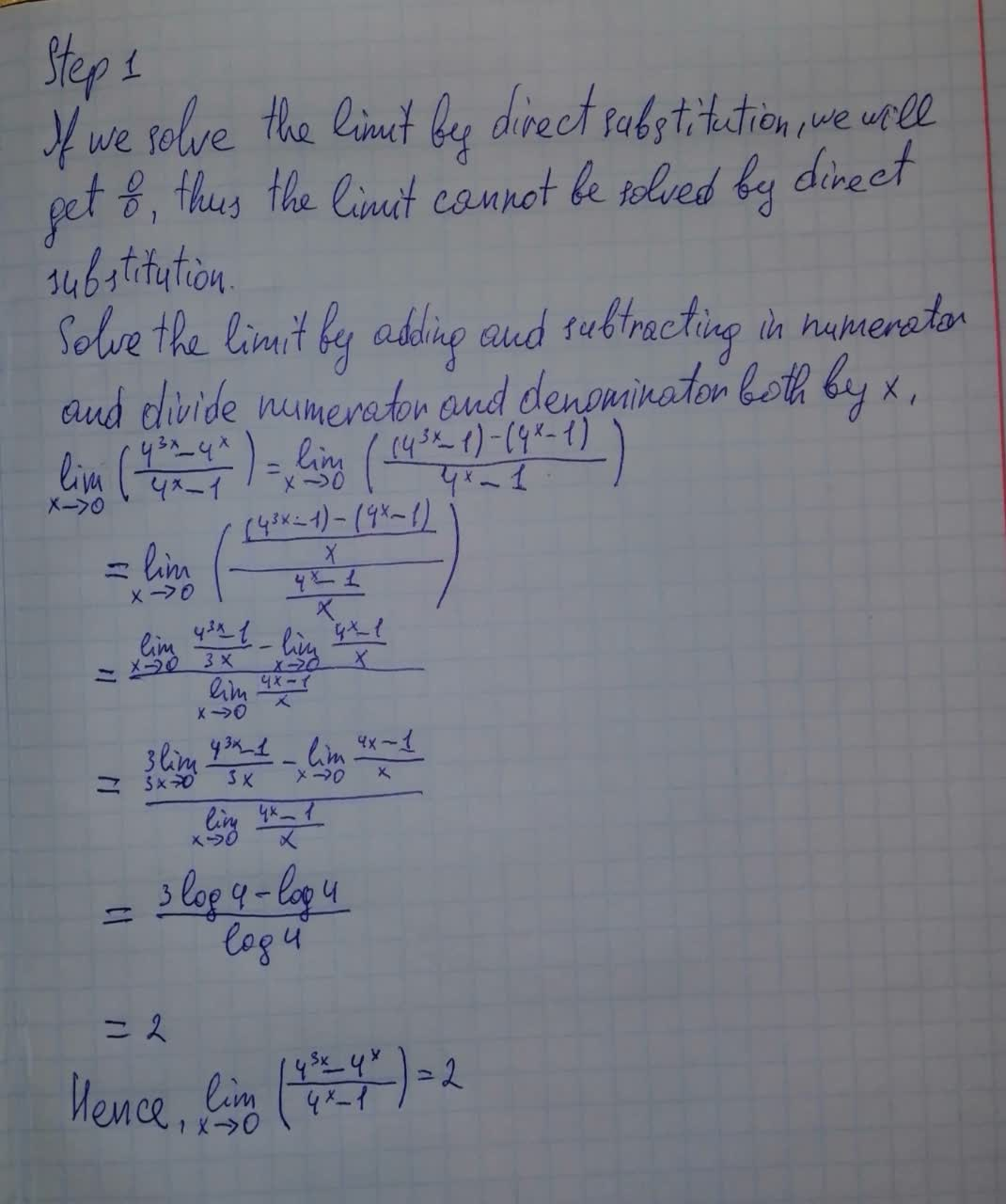# To find:The value of lim_{x \rightarrow 0} (\frac{4^{3x}-4^x}{4^x-1}) if it exists. If limit does not exist ,then find out if one-sided limit existspermaneceerc 2021-08-06 Answered
To find:
The value of $\underset{x\to 0}{lim}\left(\frac{{4}^{3x}-{4}^{x}}{{4}^{x}-1}\right)$ if it exists. If limit does not exist , then find out if one-sided limit exists.You can still ask an expert for help

• Questions are typically answered in as fast as 30 minutes

Solve your problem for the price of one coffee

• Math expert for every subject
• Pay only if we can solve itberggansS
To find the value :